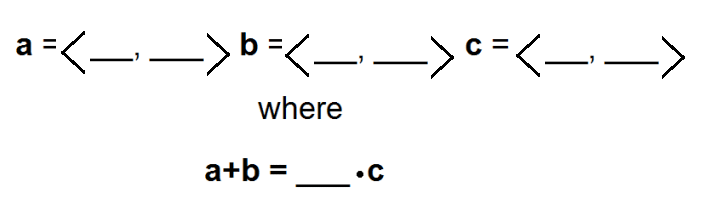Directions: Using any number between 0 and 9, fill in the boxes to create a true statement. You may only use a number once.### Hint

What are the properties of vectors? How does adding vectors relate to multiplying by a scale factor?

There are many possible solutions, one is:
<2,3>, <6,4>, <8,7> with a scaler of 1

Source: Bryan Anderson

## The Modulus Of A Complex Number

Directions: Using the digits 0 to 9 at most one time each, place a digit …

1.It could be that I am misunderstanding the question but I think the posted solution would require us to use the number 1 twice as it appears in vector a and as the scalar.

•Thank you for catching that! Yes, the original solution omitted the scaler. One possible solution would be with a scaler of 1.

I wonder if the scaler is trivial…

•I like the scalar personally … it brings one more of the 9 digits into play … I wonder if there is a solution with a scalar that isn’t 1? I don’t have time to play right now.

•Two solutions with scalars > 1
a=, b=, c=, scalar=7
a=, b=, c=, scalar=8
First digits of a and b are interchangeable, as are the second digits.

•with scaler 2
a=(6,7) and b=(4,9) for a c=(5,8)